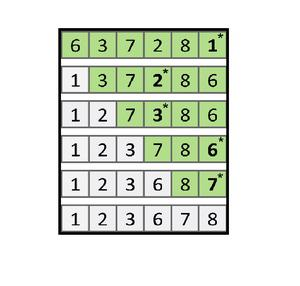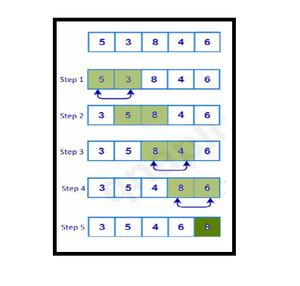# Selection Sort VS Bubble Sort

• Difficulty Level : Basic
• Last Updated : 29 Jun, 2022

Not a valid contributionIn this, we will cover the comparison between Selection Sort VS Bubble Sort. The resources required by Selection Sort & Bubble Sort algorithms on the basis of Time and Space Complexity are as follows.

`Time Complexity -Space Complexity -`

Let’s dive deep into the working of these algorithms.

Selection Sort :
The selection sort algorithm generally is the first sorting algorithm that is taught to us. Here in every iteration of the inner loop, the smallest element is replaced with the starting element in each loop. After the end of each loop, we increment the starting position by 1 and run it till the second last element in the array. Hence, by doing so at the end of the outer loop we will be having a sorted array.

The image below explains the iteration of Selection Sort Algorithm.Here we can simplify the selection sort algorithm by saying that the sorting here is done on the basis of the smallest to the largest element. The smallest element is first sorted and then the second smallest element and so on.

Implementation of Selection Sort :

Below is the implementation of the above-explained algorithm.

## C++

 `#include ``using` `namespace` `std;``void` `Selection_Sort(``int` `arr[], ``int` `n) ``{``    ``for``(``int` `i = 0; i < n - 1; ++i) ``    ``{``        ``int` `min_index = i; ``        ``for``(``int` `j = i + 1; j < n; ++j) ``        ``{``            ``if``(arr[j] < arr[min_index]) ``                ``min_index = j;``        ``}``        ``swap(arr[i], arr[min_index]); ``    ``}``}``int` `main()``{``    ``int` `n = 5;``    ``int` `arr = {2, 0, 1, 4, 3};``    ``Selection_Sort(arr, n);``    ``cout<<``"The Sorted Array by using Selection Sort is : "``;``    ``for``(``int` `i = 0; i < n; ++i)``        ``cout<

## Java

 `class` `GFG{` `  ``static` `void` `Selection_Sort(``int` `arr[], ``int` `n) ``  ``{``    ``for``(``int` `i = ``0``; i < n - ``1``; ++i) ``    ``{``      ``int` `min_index = i; ``      ``for``(``int` `j = i + ``1``; j < n; ++j) ``      ``{``        ``if``(arr[j] < arr[min_index]) ``          ``min_index = j;``      ``}``      ``int` `temp = arr[i];``      ``arr[i] = arr[min_index];``      ``arr[min_index] = temp;``    ``}``  ``}` `  ``// Driver code``  ``public` `static` `void` `main(String[] args)``  ``{``    ``int` `n = ``5``;``    ``int` `arr[] = {``2``, ``0``, ``1``, ``4``, ``3``};``    ``Selection_Sort(arr, n);``    ``System.out.print(``"The Sorted Array by using Selection Sort is : "``);``    ``for``(``int` `i = ``0``; i < n; ++i)``      ``System.out.print(arr[i] + ``" "``);``  ``}``}` `// This code is contributed by aashish1995`

## Python3

 `def` `Selection_Sort(arr, n):``    ` `    ``for` `i ``in` `range``(n ``-` `1``):``        ``min_index ``=` `i ``        ` `        ``for` `j ``in` `range``(i ``+` `1``, n):``            ``if` `(arr[j] < arr[min_index]):``                ``min_index ``=` `j``                ` `        ``arr[i], arr[min_index] ``=` `arr[min_index], arr[i] ``        ` `# Driver Code``n ``=` `5``arr ``=` `[ ``2``, ``0``, ``1``, ``4``, ``3` `]``Selection_Sort(arr, n)` `print``(``"The Sorted Array by using "` `\``      ``"Selection Sort is : "``, end ``=` `'')``for` `i ``in` `range``(n):``    ``print``(arr[i], end ``=` `" "``)``    ` `# This code is contributed by SHUBHAMSINGH10`

## C#

 `using` `System;` `public` `class` `GFG{` `  ``static` `void` `Selection_Sort(``int` `[]arr, ``int` `n) ``  ``{``    ``for``(``int` `i = 0; i < n - 1; ++i) ``    ``{``      ``int` `min_index = i; ``      ``for``(``int` `j = i + 1; j < n; ++j) ``      ``{``        ``if``(arr[j] < arr[min_index]) ``          ``min_index = j;``      ``}``      ``int` `temp = arr[i];``      ``arr[i] = arr[min_index];``      ``arr[min_index] = temp;``    ``}``  ``}` `  ``// Driver code``  ``public` `static` `void` `Main(String[] args)``  ``{``    ``int` `n = 5;``    ``int` `[]arr = {2, 0, 1, 4, 3};``    ``Selection_Sort(arr, n);``    ``Console.Write(``"The Sorted Array by using Selection Sort is : "``);``    ``for``(``int` `i = 0; i < n; ++i)``      ``Console.Write(arr[i] + ``" "``);``  ``}``}` `// This code is contributed by aashish1995`

## Javascript

 ``

Output

`The Sorted Array by using Selection Sort is : 0 1 2 3 4 `

Bubble Sort :
The bubble sort algorithm might look a little bit confusing when we first study it. But here is the easy explanation of it. Here swapping is carried on in two ways. In every iteration of the outer loop, the largest element is found and swapped with the last element in the loop. In the inner loop, we do pairwise swapping of two consecutive elements. In every inner loop, we go from the first element to the one less element we went in the previous loop. The image below shows the 1st iteration of the inner loop in the Bubble Sort Algorithm.Here we can simplify the bubble sort algorithm by saying that the sorting here is done on the basis of the largest to the smallest element. The largest element is first kept in the last location in the array. Then the second largest element in the second last location as so on.

Implementation of Bubble Sort :
Below is the implementation of the above-explained algorithm.

## C++

 `#include ``using` `namespace` `std;``void` `Bubble_Sort(``int` `arr[], ``int` `n) ``{``    ``for``(``int` `i = 1; i < n; ++i)     ``    ``{   ``                ``for``(``int` `j = 0; j <= (n - i - 1); ++j)  ``        ``{   ``            ``if``(arr[j] > arr[j + 1])``                ``swap(arr[j], arr[j + 1]); ``        ``}``    ``}``}` `int` `main()``{``    ``int` `n = 5;``    ``int` `arr = {2, 0, 1, 4, 3};``    ``Bubble_Sort(arr, n);``    ``cout<<``"The Sorted Array by using Bubble Sort is : "``;``    ``for``(``int` `i = 0; i < n; ++i)``        ``cout<

## Java

 `import` `java.io.*;` `class` `GFG{``    ` `static` `void` `Bubble_Sort(``int` `arr[], ``int` `n) ``{``    ``for``(``int` `i = ``1``; i < n; ++i)     ``    ``{``        ``for``(``int` `j = ``0``; j <= (n - i - ``1``); ++j)  ``        ``{   ``            ``if` `(arr[j] > arr[j + ``1``])``            ``{``                ``int` `temp = arr[j];``                ``arr[j] = arr[j + ``1``];``                ``arr[j + ``1``] = temp;``            ``} ``        ``}``    ``}``}` `// Driver code``public` `static` `void` `main(String[] args)``{``    ``int` `n = ``5``;``    ``int` `arr[] = { ``2``, ``0``, ``1``, ``4``, ``3` `};``    ` `    ``Bubble_Sort(arr, n);``    ` `    ``System.out.print(``"The Sorted Array by using Bubble Sort is : "``);``    ``for``(``int` `i = ``0``; i < n; ++i)``        ``System.out.print(arr[i]+``" "``);``}``}` `// This code is contributed by Shubhamsingh10`

## Python3

 `def` `Bubble_Sort(arr, n):``    ``for` `i ``in` `range``(``1``, n):``        ``for` `j ``in` `range``(``0``, n ``-` `i):``            ``if` `(arr[j] > arr[j ``+` `1``]):``                ``arr[j], arr[j ``+` `1``] ``=` `arr[j ``+` `1``], arr[j]``                ` `    ``return` `arr``    ` `# Driver Code``n ``=` `5``arr ``=` `[ ``2``, ``0``, ``1``, ``4``, ``3` `]``arr ``=` `Bubble_Sort(arr, n)` `print``(``"The Sorted Array by using Bubble Sort is : "``, end ``=` `'')``for` `i ``in` `range``(n):``    ``print``(arr[i], end ``=` `" "``)` `# This code is contributed by Shubhamsingh10`

## C#

 `// C# program for the above approach``using` `System;` `public` `class` `GFG{``    ` `    ``static` `void` `Bubble_Sort(``int``[] arr, ``int` `n) ``    ``{``        ``for``(``int` `i = 1; i < n; ++i)     ``        ``{``            ``for``(``int` `j = 0; j <= (n - i - 1); ++j)  ``            ``{   ``                ``if` `(arr[j] > arr[j + 1])``                ``{``                    ``int` `temp = arr[j];``                    ``arr[j] = arr[j + 1];``                    ``arr[j + 1] = temp;``                ``} ``            ``}``        ``}``    ``}``    ` `    ``// Driver Code``    ``static` `public` `void` `Main ()``    ``{``        ``int` `n = 5;``        ``int``[] arr = { 2, 0, 1, 4, 3 };``        ` `        ``Bubble_Sort(arr, n);``        ` `        ``Console.Write(``"The Sorted Array by using Bubble Sort is : "``);``        ``for``(``int` `i = 0; i < n; ++i){``            ``Console.Write(arr[i]+``" "``);``        ``}``    ``}``}` `// This code is contributed by Shubhamsingh10`

## Javascript

 ``

Output

`The Sorted Array by using Bubble Sort is : 0 1 2 3 4 `

1. We must account for the fact that even if our data is in sorted form initially, our current algorithm will perform all the iterations.
2. As shown by above code, we swap two elements (say i and i+1) when arr[i] > arr[i+1]. Therefore, even if our data is sorted already (or is sorted just after few iterations) our algorithm will still run,
3. However, we can tweak our code so that our algorithm recognizes when given data is sorted and no further iterations are required.

4. We can achieve this by simply adding a “flag” variable. Initialize this “flag” variable to false outside inner loop and set it to true if at any point ( arr[j] > arr[j+1] ) condition is true.
5. After inner loop is exited, check flag. If flag == true i.e, it was changed and swap operation was carried out. However, if flag == false, it means that no swap was carried out for entire iteration and hence our data is now sorted and no further iterations are required.

## C++

 `// C++ program for the above approach``#include ``using` `namespace` `std;` `// Function for bubble sort``void` `Bubble_Sort(``int` `arr[], ``int` `n)``{``    ``bool` `flag;``  ` `    ``// Iterate from 1 to n - 1``    ``for` `(``int` `i = 1; i < n; ++i) {` `        ``flag = ``false``;``      ` `        ``// Iterate from 0 to n - i - 1``        ``for` `(``int` `j = 0; j <= (n - i - 1); ++j) {``            ``if` `(arr[j] > arr[j + 1]) {``                ``swap(arr[j], arr[j + 1]);``                ``flag = ``true``;``            ``}``        ``}``        ``if` `(flag == ``false``)``            ``break``;``    ``}``}` `// Driver Code``int` `main()``{``    ``int` `n = 5;``    ``int` `arr = { 2, 0, 1, 4, 3 };``    ``Bubble_Sort(arr, n);``    ``cout << ``"The Sorted Array by using Bubble Sort is : "``;``    ``for` `(``int` `i = 0; i < n; ++i)``        ``cout << arr[i] << ``" "``;``    ``return` `0;``}`

## Java

 `// Java program for the above approach``import` `java.io.*;` `class` `GFG{``    ` `// Function for bubble sort``static` `void` `Bubble_Sort(``int``[] arr, ``int` `n)``{``    ``boolean` `flag;``  ` `    ``// Iterate from 1 to n - 1``    ``for``(``int` `i = ``1``; i < n; ++i)``    ``{``        ``flag = ``false``;``      ` `        ``// Iterate from 0 to n - i - 1``        ``for``(``int` `j = ``0``; j <= (n - i - ``1``); ++j)``        ``{``            ``if` `(arr[j] > arr[j + ``1``])``            ``{``                ``int` `temp  = arr[j];``                ``arr[j] = arr[j + ``1``];``                ``arr[j + ``1``] = temp;``                ``flag = ``true``;``            ``}``        ``}``        ``if` `(flag == ``false``)``            ``break``;``    ``}``}` `// Driver Code``public` `static` `void` `main(String[] args)``{``    ``int` `n = ``5``;``    ``int``[] arr = { ``2``, ``0``, ``1``, ``4``, ``3` `};``    ``Bubble_Sort(arr, n);``    ``System.out.print(``"The Sorted Array by "` `+``                     ``"using Bubble Sort is : "``);``    ` `    ``for``(``int` `i = ``0``; i < n; ++i)``        ``System.out.print(arr[i] + ``" "``);``}``}` `// This code is contributed by shubhamsingh10`

## Python3

 `# Python3 program for the above approach` `# Function for bubble sort``def` `Bubble_Sort(arr, n):``    ``flag ``=` `True``    ` `    ``# Iterate from 1 to n - 1``    ``for` `i ``in` `range``(``1``,n):``        ``flag ``=` `False``        ``# Iterate from 0 to n - i - 1``        ``for` `j ``in` `range``(n``-``i):``            ``if` `(arr[j] > arr[j ``+` `1``]):``                ``arr[j], arr[j ``+` `1``] ``=` `arr[j ``+` `1``], arr[j]``                ``flag ``=` `True``        ` `        ``if` `(flag ``=``=` `False``):``            ``break``        ` `# Driver Code``n ``=` `5``arr ``=` `[``2``, ``0``, ``1``, ``4``, ``3``]``Bubble_Sort(arr, n)``print``(``"The Sorted Array by using Bubble Sort is : "``, end``=``'')``for` `i ``in` `range``(n):``    ``print``(arr[i], end``=` `" "``)` `# This code is contributed by ShubhamSingh10`

## C#

 `// C# program for the above approach``using` `System;``public` `class` `GFG{``    ` `    ``// Function for bubble sort``    ``static` `void` `Bubble_Sort(``int``[] arr, ``int` `n)``    ``{``        ``bool` `flag;``      ` `        ``// Iterate from 1 to n - 1``        ``for` `(``int` `i = 1; i < n; ++i) {``    ` `            ``flag = ``false``;``          ` `            ``// Iterate from 0 to n - i - 1``            ``for` `(``int` `j = 0; j <= (n - i - 1); ++j) {``                ``if` `(arr[j] > arr[j + 1]) {``                    ``int` `temp  = arr[j];``                    ``arr[j] = arr[j + 1];``                    ``arr[j + 1] = temp;``                    ``flag = ``true``;``                ``}``            ``}``            ``if` `(flag == ``false``)``                ``break``;``        ``}``    ``}``    ` `    ``// Driver Code``    ``static` `public` `void` `Main ()``    ``{``        ``int` `n = 5;``        ``int``[] arr = { 2, 0, 1, 4, 3 };``        ``Bubble_Sort(arr, n);``        ``Console.Write(``"The Sorted Array by using Bubble Sort is : "``);``        ``for` `(``int` `i = 0; i < n; ++i)``            ``Console.Write(arr[i] + ``" "``);``    ``}` `}` `// This code is contributed by shubhamsingh10.`

## Javascript

 ``

Output

`The Sorted Array by using Bubble Sort is : 0 1 2 3 4 `

NOTE: This little tweak doesn’t change the worst case time complexity of the bubble sort algorithm but can improve its run time for particular cases.

Let us see the differences between Bubble Sort and Selection Sort in a tabular form -:

References :